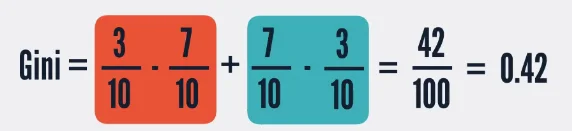05 Aug 2023

Posted on:

07 Dec 2022

0

# In Gini calculation formula at time 5:13 instead of multiplication '*' printed as subtraction '-'

Seems to be printed as subtraction instead of multiplication in below Gini Calculation formula